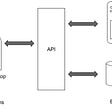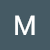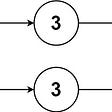# [Leet Code] Calculate Money in Leetcode Bank

`Input: n = 4Output: 10Explanation: After the 4th day, the total is 1 + 2 + 3 + 4 = 10.`
`Input: n = 10Output: 37Explanation: After the 10th day, the total is (1 + 2 + 3 + 4 + 5 + 6 + 7) + (2 + 3 + 4) = 37. Notice that on the 2nd Monday, Hercy only puts in \$2.`
`Input: n = 20Output: 96Explanation: After the 20th day, the total is (1 + 2 + 3 + 4 + 5 + 6 + 7) + (2 + 3 + 4 + 5 + 6 + 7 + 8) + (3 + 4 + 5 + 6 + 7 + 8) = 96.`
`class Solution(object):    def totalMoney(self, n):        """        :type n: int        :rtype: int        """        counter = 1        acc = 0        value = 1        starting_value = 1        while counter != n+1:            if counter % 7==0:                acc+=value                counter+=1                starting_value+=1                value=starting_value                        else:                acc+=value                value+=1                counter+=1                        return acc`

--

--

--

Learning, and posting my findings!

Love podcasts or audiobooks? Learn on the go with our new app.

## Keeping it simple: Embedded IoT connected devices## 33. Alpha Test is coming soon## Using Ghidra to read redacted PDFs## Designing a dynamic NFT with static JPEGs on IPFS## How to Deploy a Static Website using Github## Game Development in Ruby Using Gosu## What a non techie should know about APIs (Part I)## Kiwicode startup story(9): Customers need to build complex apps, not simple ones.## Matthew Boyd

Learning, and posting my findings!

## Redirecting standard input output to a file in python## LeetCode 1721: Swapping Nodes in a Linked List